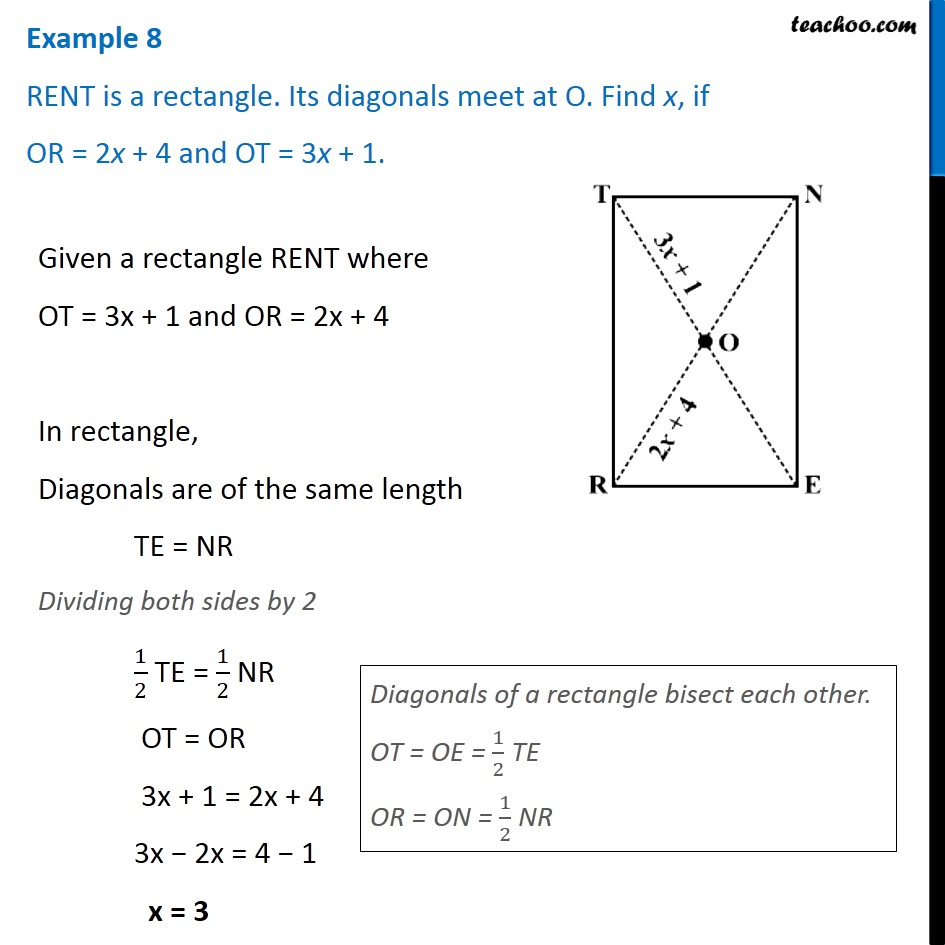Examples

Chapter 3 Class 8 Understanding Quadrilaterals
Serial order wise### Transcript

Example 8 RENT is a rectangle. Its diagonals meet at O. Find x, if OR = 2x + 4 and OT = 3x + 1.Given a rectangle RENT where OT = 3x + 1 and OR = 2x + 4 In rectangle, Diagonals are of the same length TE = NR Dividing both sides by 2 1/2 TE = 1/2 NR OT = OR 3x + 1 = 2x + 4 3x − 2x = 4 − 1 x = 3 Diagonals of a rectangle bisect each other. OT = OE = 1/2 TE OR = ON = 1/2 NR